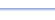Program: GCFLCM

GCFLCM

• Finds the greatest common factor (GCF) and least common multiple (LCM) of any pair of positive numbers.

Useful for:

• SAT
• ACT
• Basic Math
• Algebra 1
• Algebra 2

See full list of programsExample

Q: Find the greatest common factor and least common multiple for the numbers 45 and 80.

Solution:

Run GCFLCM.  How?

Enter the numbers
45 and 80, pressing
ENTER after each one.

The program reports:

Interpretation:

The greatest common factor is 5.
The least common multiple is 720.